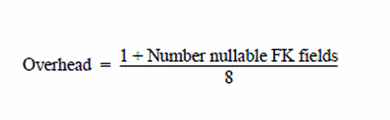# 17.10 - Sizing a Reference Index Subtable - Advanced SQL Engine - Teradata Database

## Teradata Vantage™ - Database Design

Product
Release Number
17.10
Published
July 2021
Language
English (United States)
Last Update
2021-07-27
dita:mapPath
kko1591750222108.ditamap
dita:ditavalPath
kko1591750222108.ditaval

A reference index is an internal structure that the system creates whenever a referential integrity constraint is defined between tables using a PRIMARY KEY or UNIQUE constraint on the parent table in the relationship and a REFERENCES constraint on a foreign key in the child table.

The index subtable row contains a count of the number of references in the child, or foreign key, table to the PRIMARY KEY or UNIQUE constraint in the parent table.

A maximum of 64 referential constraints can be defined for a table.

Similarly, a maximum of 64 other tables can reference a single table. Therefore, there is a maximum of 128 reference indexes that can be stored in the table header per table.

The limit on reference indexes in the table header includes both references to and from the table and is derived from 64 references to other tables plus 64 references from other tables to the current table = 128 reference index descriptors.

However, the maximum number of reference indexes stored in the reference index subtable for a table is limited to 64, defining only the relationships between the table as a parent with its child tables.

Estimate the size of your reference indexes using the equation provided below.

## Reference Index Sizing Equation

RI Subtable Size = (NumDistinct) × (FKLength + PresenceBitsOverhead + VarLengthOverhead + 25)

If fallback is defined for the child table in the relationship, then double the calculated result.

The following parameter definitions are used with this equation.

Parameter                                                     Definition
Row Count * 4 A count of the number of foreign key row references is stored in a reference index subtable. Each foreign key row count is 4 bytes long.
FKLength The length of a fixed length foreign key value in bytes.

Use one of these parameters depending on the reference index in question.

• If the FK column value is fixed, then use the length of the value.
• If the FK column value is variable, then use the average length of the variable length Foreign Key values.
NumDistinct The value is an estimate of the number of distinct foreign key subtable values.

Exclude null foreign keys from this estimate.

Presence Bits Overhead Use the following equation to calculate this parameter:If there are no presence bits, then the value for this parameter is 0.

VarLength Overhead Use the following equation to calculate this parameter:

Overhead = 2 × (Number variable length FK fields + 1)

If there are no variable length foreign keys, then the value for this parameter is 0.

RI Block Overhead Sum of the following factors.
• Row length
• RI row rowID
• Spare byte
• Presence octets
• Offsets
• Valid flag
• Foreign key count
• Reference array

= 25 bytes